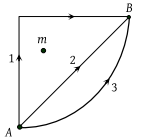If W1, W2 and W3 represent the work done in moving a particle from A to B along three different paths 1, 2 and 3 respectively (as shown) in the gravitational field of a point mass m, find the correct relation between W1, W2 and W3(1) W1 > W2 > W3

(2) W1 = W2 = W3

(3) W1 < W2 < W3

(4) W2 > W1 > W3

Concept Questions :-

Gravitational Potential energy
High Yielding Test Series + Question Bank - NEET 2020

Difficulty Level:

A particle of mass m is moving in a horizontal circle of radius r under a centripetal force equal to –K/r2, where K is a constant. The total energy of the particle is

1. $\frac{K}{2r}$

2. $-\frac{K}{2r}$

3. $-\frac{K}{r}$

4. $\frac{K}{r}$

Concept Questions :-

Potential energy: Relation with force
High Yielding Test Series + Question Bank - NEET 2020

Difficulty Level:

The displacement x of a particle moving in one dimension under the action of a constant force is related to the time t by the equation $t=\sqrt{x}+3$, where x is in meters and t is in seconds. The work done by the force in the first 6 seconds is

(1) 9 J

(2) 6 J

(3) 0 J

(4) 3 J

Concept Questions :-

Concept of work
High Yielding Test Series + Question Bank - NEET 2020

Difficulty Level:

A force $\mathbit{F}=-K\left(y\mathbit{i}+x\mathbit{j}\right)$ (where K is a positive constant) acts on a particle moving in the xy-plane. Starting from the origin, the particle is taken along the positive x-axis to the point (a, 0) and then parallel to the y-axis to the point (a, a). The total work done by the force F on the particles is

(1) $-2K{a}^{2}$

(2) $2K{a}^{2}$

(3) $-K{a}^{2}$

(4) $K{a}^{2}$

Concept Questions :-

Work done by variable force
High Yielding Test Series + Question Bank - NEET 2020

Difficulty Level:

If g is the acceleration due to gravity on the earth's surface, the gain in the potential energy of an object of mass m raised from the surface of earth to a height equal to the radius of the earth R, is

(1) $\frac{1}{2}mgR$

(2) 2 mgR

(3) mgR

(4) $\frac{1}{4}mgR$

Concept Questions :-

Gravitational Potential energy
High Yielding Test Series + Question Bank - NEET 2020

Difficulty Level:

A lorry and a car moving with the same K.E. are brought to rest by applying the same retarding force, then

(1) Lorry will come to rest in a shorter distance

(2) Car will come to rest in a shorter distance

(3) Both come to rest in a same distance

(4) None of the above

Concept Questions :-

Concept of work
High Yielding Test Series + Question Bank - NEET 2020

Difficulty Level:

A particle free to move along the x-axis has potential energy given by $U\left(x\right)=k\left[1-{e}^{{\left(-x\right)}^{2}}\right]$ for $-\infty \le x\le +\infty$, where k is a positive constant of appropriate dimensions. Then

(1) At point away from the origin, the particle is in unstable equilibrium

(2) For any finite non-zero value of x, there is a force directed away from the origin

(3) If its total mechanical energy is k/2, it has its minimum kinetic energy at the origin

(4) For small displacements from x = 0, the motion is simple harmonic

Concept Questions :-

Potential energy: Relation with force
High Yielding Test Series + Question Bank - NEET 2020

Difficulty Level:

The kinetic energy acquired by a mass m in travelling a certain distance d starting from rest under the action of a constant force is directly proportional to

(1) $\sqrt{m}$

(2) Independent of m

(3) $1/\sqrt{m}$

(4) m

Concept Questions :-

Concept of work
High Yielding Test Series + Question Bank - NEET 2020

Difficulty Level:

An open knife edge of mass 'm' is dropped from a height 'h' on a wooden floor. If the blade penetrates upto the depth 'd' into the wood, the average resistance offered by the wood to the knife edge is

(1) mg

(2) $mg\left(1-\frac{h}{d}\right)$

(3) $mg\left(1+\frac{h}{d}\right)$

(4) $mg{\left(1+\frac{h}{d}\right)}^{2}$

Concept Questions :-

Gravitational Potential energy
High Yielding Test Series + Question Bank - NEET 2020

Difficulty Level:

A body is moving along a straight line by a machine delivering constant power. The distance moved by the body in time t is proportional to

(1) t1/2

(2) t3/4

(3) t3/2

(4) t2

Concept Questions :-

Power